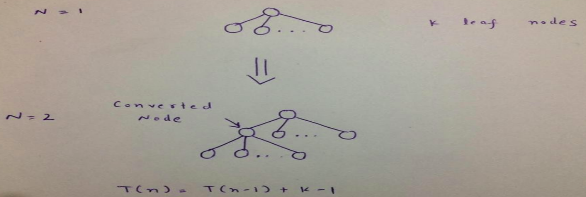GATE | GATE-CS-2005 | Question 36

• Difficulty Level : Easy
• Last Updated : 28 Jun, 2021

In a complete k-ary tree, every internal node has exactly k children. The number of leaves in such a tree with n internal nodes is
(A) nk
(B) (n – 1)k + 1
(C) n(k – 1) + 1
(D) n(k – 1)

Explanation:
Background required – Trees and Recurrence Relation

We have to tackle this problem by forming a recurrence relation. Let T(n) be number of leaves in a tree having n internal nodes. We have to somehow relate T(n) to T(n-1).

Consider a tree with n = 1. This tree will be having exactly k Leaves.

Try creating a tree with 2 internal nodes from the above tree. We have to make one leaf node an internal node and while doing this, we are getting more k leaves. Therefore, number of leaf nodes in the newly constructed tree will be one less than original tree as we have changed one leaf to internal node plus k (Newly converted node has now spawn k more leaves).Thus, T(n)   = T(n-1) - 1 + k = T(n-1) + k -1
= T(n-2) + 2*(k-1)         Solving By Substitution Method
= T(n-3) + 3*(k-1)
.
.
.
= T(n-(n-1)) + (n-1)*(k-1)
= T(1) + (n-1)*(k-1)
= k + (n-1)*(k-1)             Forming Base Case : T(1) = k
= n(k – 1) + 1

In Gate, we will suggest you to solve this question by taking 2-3 examples and eliminating the options. But in the interviews later, you might have to show them how you form and solve the recurrence relation.

This explanation has been contributed by Pranjul Ahuja.